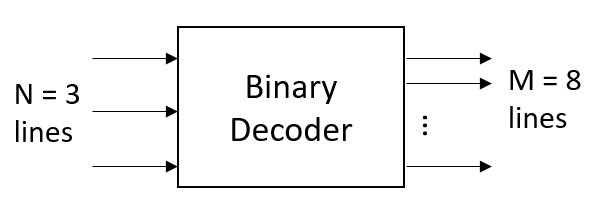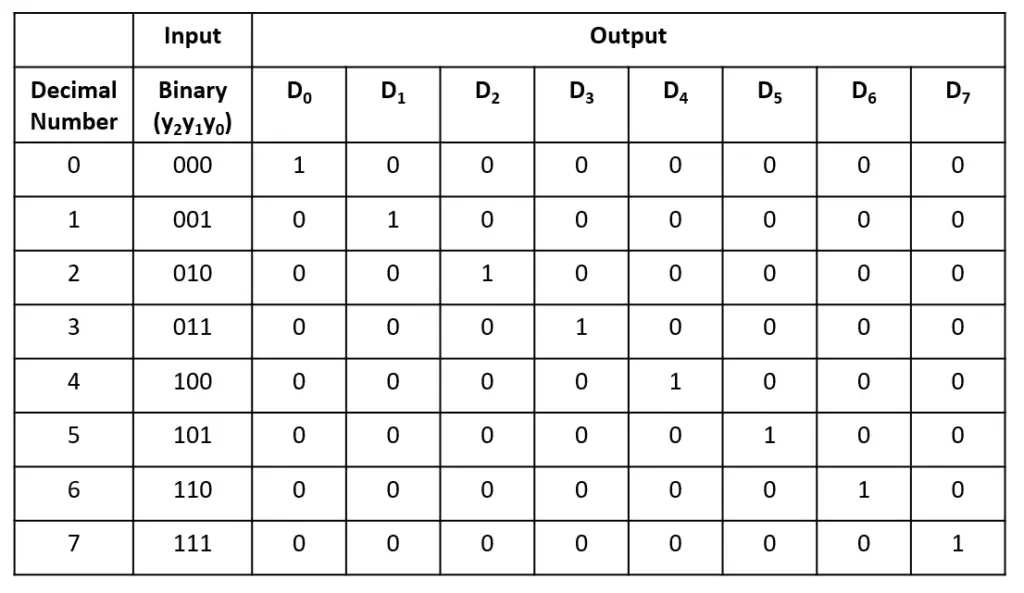Tutorials

The decoder behaves exactly opposite of the encoder. They decode already coded input to its decoded form. The ‘N’(=n) input coded lines decode to ‘M’(=2^n) decoded output lines. The decoder sets exactly one line high at the output for a given encoded input line.

Nomenclature: N: M decoder where N denotes coded input lines and M denotes decoded output lines

## 3:8 Binary decoder

The 3 input lines denote 3-bit binary code and 8 output line represents its decoded decimal form.

### Block Diagram### Truth Table### 3:8 Binary Decoder Verilog Code

``````module binary_decoder(
input [2:0] D,
output reg [7:0] y);

always@(D) begin
y = 0;
case(D)
3'b000: y = 1'b1;
3'b001: y = 1'b1;
3'b010: y = 1'b1;
3'b011: y = 1'b1;
3'b100: y = 1'b1;
3'b101: y = 1'b1;
3'b110: y = 1'b1;
3'b111: y = 1'b1;
default: y = 0;
endcase
end
endmodule``````

### Truth Table

``````module tb;
reg [2:0] D;
wire [7:0] y;

binary_decoder bin_dec(D, y);

initial begin
\$monitor("D = %b -> y = %0b", D, y);
repeat(5) begin
D=\$random; #1;
end
end
endmodule``````

Output:

``````D = 100 -> y = 10000
D = 001 -> y = 10
D = 011 -> y = 1000
D = 101 -> y = 100000``````

Verilog Codes